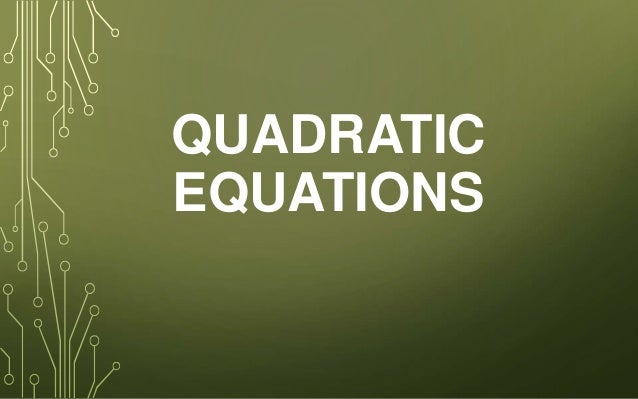Successfully reported this slideshow.
We use your LinkedIn profile and activity data to personalize ads and to show you more relevant ads. You can change your ad preferences anytime.Upcoming SlideShare
×

# Mathematics 9 Lesson 1: Quadratic Equations

551 views

Published on

This powerpoint presentation discusses or talks about the topic or lesson Quadratic Equations. It also discusses the steps in factoring or solving quadratic equations using the method of Factoring.

Published in: Education
• Full Name
Comment goes here.

Are you sure you want to Yes No
Your message goes here• Be the first to comment

### Mathematics 9 Lesson 1: Quadratic Equations

1. 1. QUADRATIC EQUATIONS
2. 2. QUADRATIC EQUATIONS •It is an equation of a degree 2 •Standard form of Quadratic Equation: ax2 + bx + c where a, b and c are real numbers, and a ≠0
3. 3. STEPS IN SOLVING QUADRATIC EQUATIONS BY FACTORING 1. Transpose all terms on the left side of the equation, if necessary 2. Combine similar terms 3. Factor the left side of the equation 4. Equate each factor to zero 5. Solve the equation in step in 4 6. Check each root by substituting it in the original equation
4. 4. SOLVING QUADRATIC EQUATIONS BY FACTORING Example: x2 – 8x – 0 Step 1: x2 – 8x – 0 (Factor) Step 2: x(x-8) – 0 (Equate each factor to 0) Step 3: x-0 or x-8 = 0 (Add 8 to both sides) x = 8